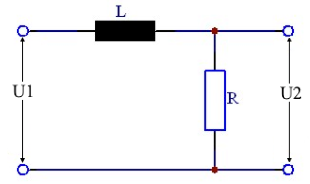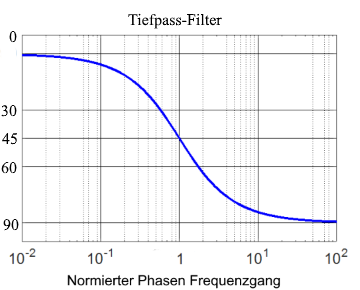# RL low pass filter

Calculator and formulas for calculating the parameters of an RL low pass filter

## RL low pass filter calculator

This function calculates the properties of a low-pass filter consisting of a resistor and a coil. The output voltage, attenuation and phase shift are calculated for the given frequency.

RL low pass filter calculator

 Input Resistor mΩ Ω kΩ MΩ Coil H mH µH nH Frequency Hz kHz MHz GHz Input voltage µV mV V kV Decimal places 0 1 2 3 4 6 8 10 Results Reactance XL Ouput voltage U2 Attenuation dB Phase shift φ$$\displaystyle L$$ = Inductance [H]

$$\displaystyle R$$ = Resistance [Ω]

$$\displaystyle U_1$$ = Input voltage [V]

$$\displaystyle U_2$$ = Output voltage [V]

$$\displaystyle X_L$$ = Reactance [Ω]

$$\displaystyle f_g$$ = Cutoff frequency [Hz]

$$\displaystyle φ$$ = Phase angle [°]

## Formulas for the RL low pass

### Calculate the voltage ratio

The output voltage U2 of an RL low pass is calculated using the following formula.

$$\displaystyle U_2=U_1 ·\frac{R} {\sqrt{R^2 + (2 · π · f · L)^2}}$$

or simply if XL is known

$$\displaystyle U_2=U_1 ·\frac{R}{\sqrt{R^2 + X_L^2}}$$
$$\displaystyle X_L=2 π · f ·L$$

### Attenuation in decibels

At the resonance frequency, the damping is 3 dB. It can be calculated for the various frequencies using the formulas below. If the input and output voltage are known, the attenuation can easily be calculated using the following formula.

$$\displaystyle V_u=20 · lg \left(\frac{U_2}{U_1} \right)$$

If the voltages are not known, the following formula is used.

$$\displaystyle V_u=20·lg\left(\frac{R} {\sqrt{R^2 + (2 · π · f · L)^2}}\right)$$

or simply shown

$$\displaystyle V_u=20·lg\left(\frac{R} {\sqrt{R^2 + (ω · L)^2}}\right)$$

### Phase shift

In an RL low pass, the output voltage lags behind the input voltage by 0 ° to -90 °, depending on the frequency. At the resonance frequency, the phase shift is -45 °. At low frequencies it tends towards 0. At high frequencies the phase rotates towards -90 °. The phase shift can be calculated using the following formula.

$$\displaystyle φ=acos \left(\frac{U_2}{U_1} \right)$$
$$\displaystyle φ= arctan \left(ω · \frac{L}{R}\right)$$### Cutoff frequency

At the cutoff frequency fg or ωg the value of the amplitude-frequency response (ie the magnitude of the transfer function) is 0.707, which corresponds to -3dB.

$$\displaystyle 0.707= \frac{1}{\sqrt{2}}$$

#### Cutoff frequency formulas

$$\displaystyle ω_g= \frac{R}{L} ⇒ f_g=\frac{R}{2·π·L}$$
$$\displaystyle R= 2·π·f_g·L$$
$$\displaystyle L=\frac{R}{2·π·f_g}$$